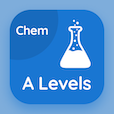Management Online Courses (MBA)

MBA Statistics MCQs

MBA Statistics MCQ PDF - Topics

# Properties of Point Estimators MCQ Quiz Online

Learn Properties of Point Estimators Multiple Choice Questions (MCQ), Properties of Point Estimators quiz answers PDF to study mba statistics online course for mba statistics classes. Descriptive Statistics Multiple Choice Questions and Answers (MCQs), Properties of Point Estimators quiz questions for easiest online MBA. "Properties of Point Estimators MCQ" PDF Book: descriptive statistics test prep for full time MBA.

"A comparison with test statistic to determine whether the null hypothesis should be rejected is called" MCQ PDF: properties of point estimators with choices critical interval, critical value, confidence coefficient, and confidence interval for easiest online MBA. Study properties of point estimators quiz questions for merit scholarship test and certificate programs .

## MCQs on Properties of Point Estimators Quiz

MCQ: A comparison with test statistic to determine whether the null hypothesis should be rejected is called

Critical interval
Critical value
Confidence coefficient
Confidence interval

MCQ: Probability sampling techniques named as systematic sampling and

Cluster sampling
Stratified sampling
Random sampling
Both A and B

MCQ: The point estimator with the smaller standard error is said to have greater

Biasness
Unbiasness
Efficiency
Consistency

MCQ: More evidences against the null hypothesis can be measured given by

Smaller p-values
Larger p-values
Narrow confidence interval
Wide confidence interval

MCQ: A measure of the evidence against the null hypothesis given by the sample is known to be as

Critical value
Test statistics
p-value
Level of significance

### More Topics from MBA Statistics Course